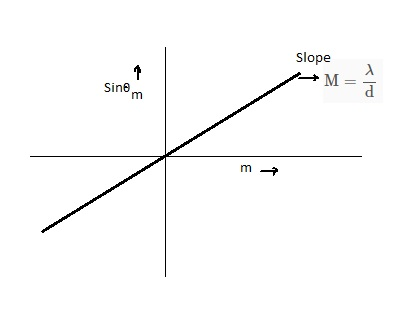# Suppose you observe a series of interference maxima and plot sin ? m vs. for those maxima. What...

## Question:

Suppose you observe a series of interference maxima and plot {eq}\sin \theta_m{/eq} vs. for those maxima. What type of graph would you expect get? Describe not only the shape of the graph but also the parameters that would describe it.

## Interference Pattern:

The interference is the phenomenon where the light superimposed after passing from the slit. When the light interferes constructively, then the bright fringes formed or we can say maxima. When the path difference between the waves is an integral multiple of the wavelength, then the maxima forms.The Path difference between the waves is given by the following expression

{eq}\rm \delta x = d\sin\theta_{m} {/eq}

where

• d is the slit width
• {eq}\rm \theta_{m} {/eq} is the angular position of the fringe

When the path difference is equal to the integral multiple of the wavelength, then the constructive interference or maxima forms, therefore

{eq}\rm \delta x = m \lambda \\ d\sin\theta_{m} = m \lambda \\ \sin\theta = \dfrac{\lambda}{d} m {/eq}

where

• m is the order of the fringe
• {eq}\rm \lambda {/eq} is the wavelength

For the plot of the {eq}\rm \sin\theta_{m} {/eq} vs {eq}\rm m {/eq}

the above equation resembles with the equation of the straight line passing through the origin i.e. {eq}\rm y = Mx {/eq}

where "M" represents the slope of the graph which would be equal to, {eq}\rm M = \dfrac{\lambda}{d} {/eq}